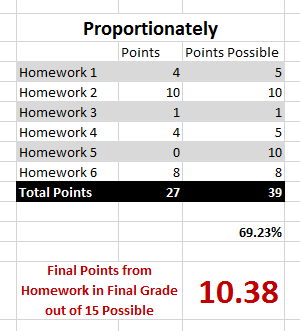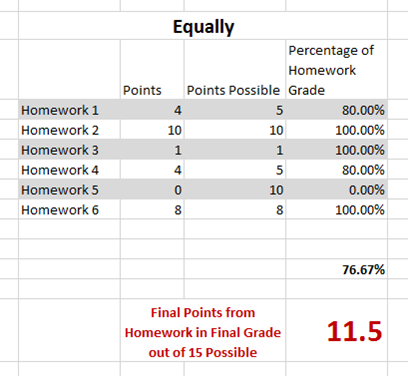## Understanding the Difference Between Equally and Proportionally Weighted Categories

It’s important to know if grades within each category are weighted equally or proportionately.

#### Weighted Proportionally:

##### For example, all of Mary’s homework assignments are worth 15 percent or 15 points out of 100 of her grade.

Homework 1: 4/5

Homework 2: 10/10

Homework 3: 1/1

Homework 4: 4/5

Homework 5: 0/10

Homework 6: 8/8

If the grades are weighted proportionately, add up all of the points that she received and all of the points that were possible and divide to get her total percentage of her 15 points for homework.

In the case below, 69.23% of 15 points is 10.38 points.  This is Mary’s final points for Homework.#### Weighted Equally:

If grades within a category are weighted equally then each assignment within that category will be worth the same as every other homework assignment regardless of actual point value.

Divide each assignment points earned by each assignment points possible to get the percentage.

Next, take an average of those percentages.  That average is the percentage of the final 15 points for homework.

In the case below, 76.67% of 15 points is 11.5. This is Mary’s final points for Homework.